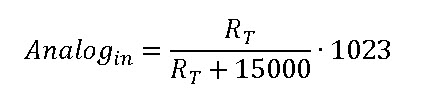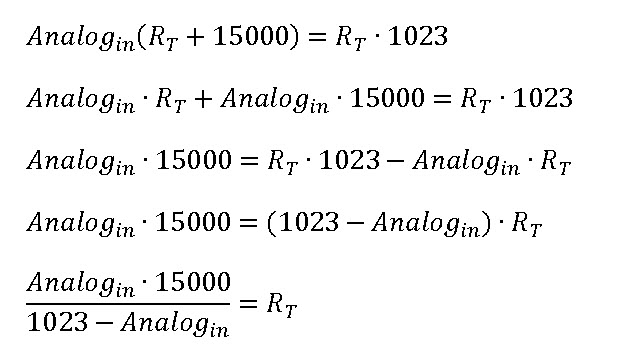# Vernier Shield Hookup Guide

Pages

## Example 4 - Temperature Probe

Measuring temperature is a quantity that crosses into nearly every area of science and engineering (except maybe computer science). Vernier sells a great stainless steel temperature probe that is accurate from -40 degrees C to +135 degrees C. According to their site, the sensor can handle up to 150 degrees C before it is damaged. For us in the U.S., this is about 300 degrees F. It's not quite safe to use as a meat thermometer in the oven, but it covers a pretty wide range for most scientific exporations.

The probe has a device called a thermistor that exhibits a change in resistance with respect to temperature. The Vernier probe uses a 20 kΩ NTC (negative thermal coefficient) Thermistor. As the temperature increases, the resistance drops.

The resistance vs. temperature relationship is non-linear and pretty complex, but it can be approximated using the Steinhart-Hart equation:

Where, RT is the resistance of the thermistor, and k0, k1, k2 are unique constants for the device. For the Vernier sensor:

``````k0 = 1.02119E-3
k1 = 2.22468E-4
k2 = 1.33342E-7
``````

You can write your own function to convert the analog read value from the Arduino to a resistance.

Schematic:

The Vernier Interface Shield has a 15k Pull-up Resistor on Vres. We can determine the resistance of the thermistor because this is a simple voltage divider circuit:So - here's the derivation of the work and the algebra:### Example Code

The example below will collect data and display it to the Serial Monitor. After uploading this example sketch to your Arduino, open up a Serial Monitor to view the data.

This example code will take measurements at a rate of 2 samples per second. Modify the variable dataRate to adjust the sampling speed.

This code has a function called resistance() that converts the raw AnalogRead value to the resistance of the thermistor, and a function called steinharthart() that applies the Steinhart-Hart equation and returns a temperature in degrees Celsius.

``````language:c
/*
VernierThermistor (v 2013.11)
Reads the temperature from a Vernier Stainless Steel Temperature Probe (TMP-BTA)
or Surface Temperature Sensor (STS-BTA) connected to the BTA connector.
As written, the readings will be displayed every half second. Change the variable

We use the Steinhart-Hart equation (in the function Thermistor) to determine temperature
from the raw A/D converter reading. Because of the use of log functions, in the Steinhart-Hart
equation, this sketch requires the math.h library.

See www.vernier.com/engineering/stem/sensors/temperature-sensor/

Modifications by B. Huang (Feb 2014)
-------------------------
Removed the use of delays in the loop() function. Uses a timeRef variable
Added a variable called dataRate. dataRate describes the # of samples per second.
Added separate functions for calculating the resistance of the thermistor and then applying the
Steinhart-hart equation.

*/

float dataRate = 2;             // set # of samples per second.
const char delimiter = '\t';  // delimitter character

const int ThermistorPIN = A0; // A0 for Analog1 and A2 for Analog 2
float Temp;

// Variables used in the code for calculations
unsigned long timeRef;    // reference for starting time

unsigned long timeInterval;
unsigned long ndx;        // index for data counter
unsigned long thermistor;

void setup()
{
Serial.begin(9600);
Serial.println("Vernier Format 2");
Serial.println("Data Set");
Serial.print("Time");
Serial.print("\t"); //tab character
Serial.println ("Temperature");
Serial.print("seconds");
Serial.print("\t"); // tab character
Serial.println ("degrees C");
timeInterval = 1000 / dataRate;  // calculates the timeInterval based on # of samples per second.
timeRef = millis();
}

void loop()
{
if ((millis()) >= ndx*timeInterval + timeRef)  // controls so only runs once per timeInterval
{
ndx++;
//the print below does the division first to avoid overflows
Serial.print((float)(millis() - timeRef) / 1000, 2);
thermistor = resistance(rawAnalogReading);     // converts raw analog value to a resistance
Temp = steinharthart(thermistor);              // Applies the Steinhart-hart equation

Serial.print(delimiter); //tab character
Serial.println(Temp,1);   // display temperature to one digit
}
}
* Schematic:
*   [Ground] -- [thermistor] -------- | -- [15,000 ohm bridge resistor] --[Vcc (5v)]
*                                     |
*                                Analog Pin 0
*
* For the circuit above:
*/
{
unsigned long temp;  // temporary variable to store calculations in
return temp; // returns the value calculated to the calling function.
}

float steinharthart(unsigned long resistance)
// function users steinhart-hart equation to return a temperature in degrees celsius.
/* Inputs ADC count from Thermistor and outputs Temperature in Celsius
* There is a huge amount of information on the web about using thermistors with the Arduino.
* Here we are concerned about using the Vernier Stainless Steel Temperature Probe TMP-BTA and the
* Vernier Surface Temperature Probe STS-BTA, but the general principles are easy to extend to other
* thermistors.
* This version utilizes the Steinhart-Hart Thermistor Equation:
*    Temperature in Kelvin = 1 / {A + B[ln(R)] + C[ln(R)]^3}
*   for the themistor in the Vernier TMP-BTA probe:
*    A =0.00102119 , B = 0.000222468 and C = 1.33342E-7
*    Using these values should get agreement within 1 degree C to the same probe used with one
*    of the Vernier interfaces
*
*/
{
float temp; // temporary variable to store calculations in
float logRes = log(resistance);
// calculating logirithms is time consuming for a microcontroller - so we just
// do this once and store it to a variable.
float k0 = 0.00102119;
float k1 = 0.000222468;
float k2 = 0.000000133342;

temp = 1 / (k0 + k1 * logRes + k2 * logRes*logRes*logRes);
temp = temp - 273.15;  // convert from Kelvin to Celsius
return temp;
}
``````

For more information on thermistors, take a look at Vernier's resource on making temperature measurements